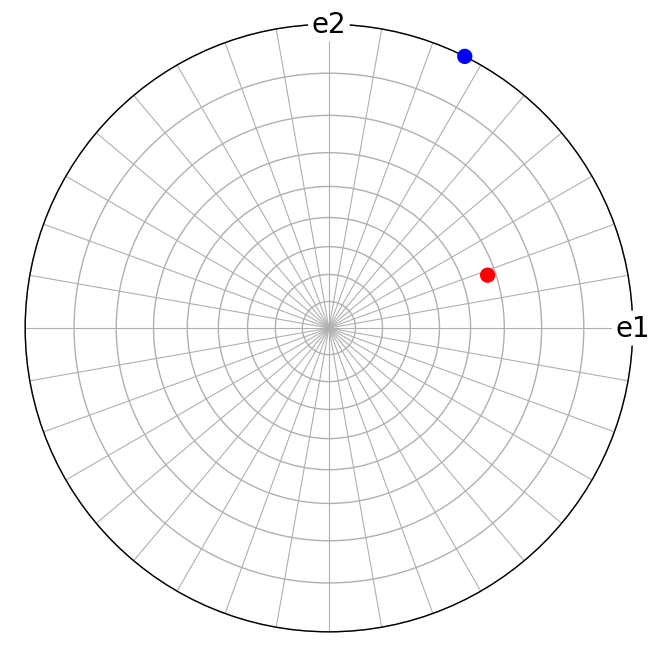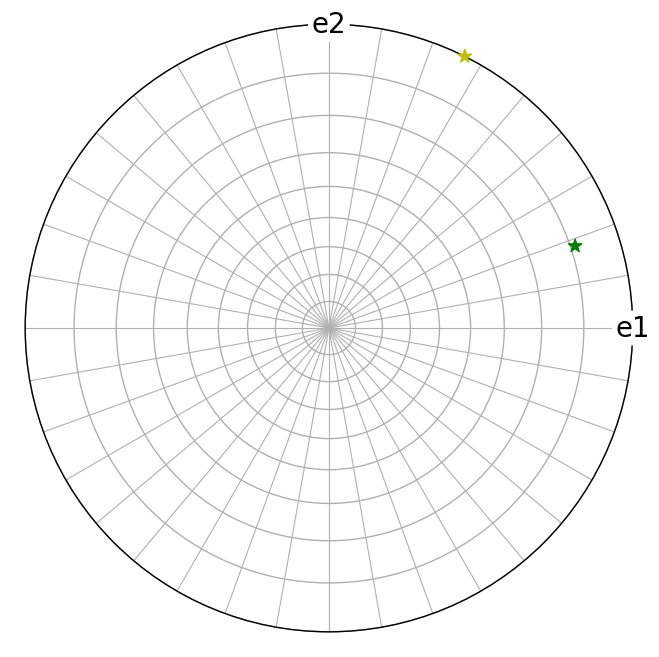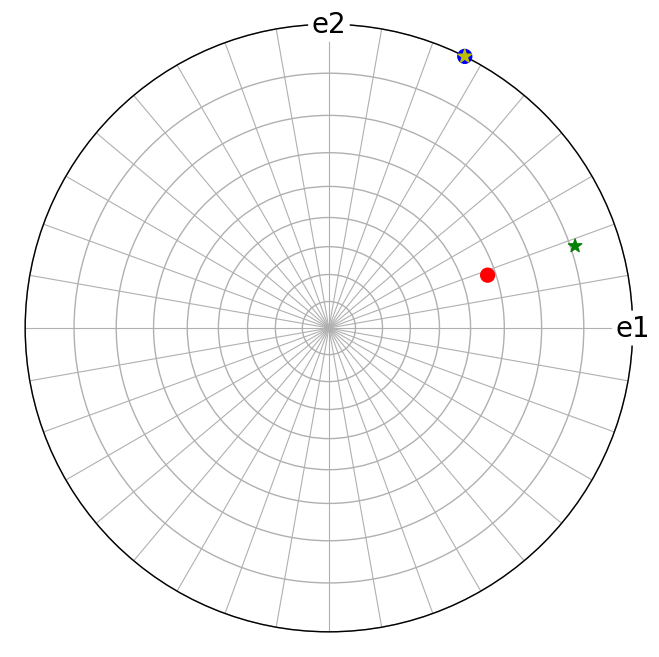# Crystal directions#

In this tutorial we will perform operations with and plot directions with resepct to the crystal reference frame using Miller indices with the orix.vector.Miller class.

Many of the initial examples, explaining basic crystallographic computations with crystal lattice directions $$[uvw]$$ and crystal lattice planes $$(hkl)$$, are taken from the textbook Introduction to Conventional Transmission Electron Microscopy (). Some of the later examples are also inspired by MTEX’ excellent documentation on Miller indices and operations with them.

:

%matplotlib inline

from diffpy.structure import Lattice, Structure
import matplotlib.pyplot as plt
import numpy as np
from orix.crystal_map import Phase
from orix.quaternion import Orientation, Rotation, symmetry
from orix.vector import Miller, Vector3d

plt.rcParams.update(
{
"figure.figsize": (7, 7),
"font.size": 20,
"axes.grid": True,
"lines.markersize": 10,
"lines.linewidth": 2,
}
)


To start with, let’s create a tetragonal crystal with lattice parameters $$a$$ = $$b$$ = 0.5 nm and $$c$$ = 1 nm and symmetry elements described by point group 4

:

tetragonal = Phase(
point_group="4",
structure=Structure(lattice=Lattice(0.5, 0.5, 1, 90, 90, 90)),
)
print(tetragonal)
print(tetragonal.structure.lattice)

<name: . space group: None. point group: 4. proper point group: 4. color: tab:blue>
Lattice(a=0.5, b=0.5, c=1, alpha=90, beta=90, gamma=90)


Here, the Phase class attaches a point group symmetry, Symmetry, to a Structure containing a Lattice (where the crystal axes are defined), and possibly some Atoms.

## Directions $$[uvw]$$#

A crystal lattice direction $$\mathbf{m} = u \cdot \mathbf{a} + v \cdot \mathbf{b} + w \cdot \mathbf{c}$$ is a vector with coordinates $$u, v, w$$ with respect to the crystal axes $$\mathbf{a}, \mathbf{b}, \mathbf{c}$$, and is denoted $$[uvw]$$. We can create a set of these Miller indices by passing a list/array/tuple to uvw in Miller

:

m1 = Miller(uvw=[[1, 2, 0], [3, 1, 1]], phase=tetragonal)
m1.scatter(axes_labels=["x", "y", None], c=["b", "r"])
m1

:

Miller (2,), point group 4, uvw
[[1. 2. 0.]
[3. 1. 1.]]Here, we plotted the lattice directions in the stereographic projection using the Vector3d.scatter() plotting method, which also works for Miller.scatter() since the Miller class inherits most of the functionality in the Vector3d class.

Let’s compute the dot product in nanometres and the angle in degrees between the vectors

:

m1.dot(m1)

:

array([1.25])

:

m1.angle_with(m1, degrees=True)

:

array([53.3007748])


The length of a direct lattice vector is available via the Miller.length property, given in lattice parameter units (nm in this case)

:

Miller(uvw=[0, -0.5, 0.5], phase=tetragonal).length

:

array([0.55901699])


## Planes $$(hkl)$$#

A crystal lattice plane $$(hkl)$$ is described by its normal vector $$\mathbf{n} = h\cdot\mathbf{a^*} + k\cdot\mathbf{b^*} + l\cdot\mathbf{c^*}$$, where $$\mathbf{a^*}, \mathbf{b^*}, \mathbf{c^*}$$ are the reciprocal crystal axes. As for crystal directions $$[uvw]$$, we can create a set of these Miller indices by passing hkl instead of uvw to Miller

:

m2 = Miller(hkl=m1.uvw, phase=tetragonal)
m2.scatter(c=["y", "g"], marker="*")
m2

:

Miller (2,), point group 4, hkl
[[1. 2. 0.]
[3. 1. 1.]]Let’s compute the angle in degrees between the lattice plane normals

:

m2.angle_with(m2, degrees=True)

:

array([45.69879831])


Note that the lattice plane normals $$(hkl)$$ are not always parallel to the lattice directions $$[uvw]$$, even if the indices are the same

:

fig = m1.scatter(return_figure=True, c=["b", "r"])
m2.scatter(figure=fig, c=["y", "g"], marker="*")We can get the reciprocal components of the lattice vector  (i.e. which lattice plane the  direction is perpendicular to) by accessing Miller.hkl for a Miller object with crystal directions (or Miller.uvw for a Miller object with crystal plane normals)

:

Miller(uvw=[1, 1, 4], phase=tetragonal).hkl

:

array([[0.25, 0.25, 4.  ]])


We see that the lattice vector  is perpendicular to the lattice plane (1 1 16).

The length of reciprocal lattice vectors can also be accessed via the Miller.length property, and equals $$\left|\mathbf{g}_{\mathrm{hkl}}\right| = \frac{1}{d_{\mathrm{hkl}}}$$ in reciprocal lattice parameter units (nm^-1 in this case), meaning we can obtain the interplanar spacing $$d_{\mathrm{hkl}}$$

:

m2.length

:

array([4.47213595, 6.40312424])

:

1 / m2.length

:

array([0.2236068 , 0.15617376])


## Zone axes#

The cross product of the lattice vectors  and  in the tetragonal crystal, in direct space, is described by a vector expressed in reciprocal space, $$(hkl)$$

:

m3 = Miller(uvw=[[1, 1, 0], [1, 1, 1]], phase=tetragonal)
m3perp = m3.cross(m3)
m3perp

:

Miller (1,), point group 4, hkl
[[ 0.25 -0.25  0.  ]]


The exact “indices” are returned, however, we can get a new Miller instance with the indices rounded down or up to the closest smallest integer via the Miller.round() method

:

m3perp.round()

:

Miller (1,), point group 4, hkl
[[ 1. -1.  0.]]


The maximum index that Miller.round() might return can be set by passing the max_index parameter.

We can plot these direct lattice vectors $$[uvw]$$ and the vectors normal to the cross product vector, i.e. normal to the reciprocal lattice vector $$(hkl)$$

:

fig = m3.scatter(return_figure=True, c="r")
m3perp.draw_circle(figure=fig, color="b", linestyle="--")Likewise, the cross product of reciprocal lattice vectors (110) and (111) results in a direct lattice vector

:

m4 = Miller(hkl=[[1, 1, 0], [1, 1, 1]], phase=tetragonal)
m4perp = m4.cross(m4).round()
m4perp

:

Miller (1,), point group 4, uvw
[[ 1. -1.  0.]]

:

fig = m4.scatter(return_figure=True, c="b")
m4perp.draw_circle(figure=fig, color="r", linestyle="--")## Trigonal and hexagonal index conventions#

Crystal lattice vectors and planes in lattices with trigonal and hexagonal crystal symmetry are typically expressed in Weber symbols $$[UVTW]$$ and Miller-Bravais indices $$(hkil)$$. The definition of $$[UVTW]$$ used in orix follows Introduction to Conventional Transmission Electron Microscopy (DeGraef, 2003)

\begin{align} U &= \frac{2u - v}{3},\\ V &= \frac{2v - u}{3},\\ T &= -\frac{u + v}{3},\\ W &= w. \end{align}

For a plane, the $$h$$, $$k$$ and $$l$$ indices are the same in $$(hkl)$$ and $$(hkil)$$, and $$i = -(h + k)$$.

The first three Miller indices always sum up to zero, i.e.

\begin{align} U + V + T &= 0,\\ h + k + i &= 0. \end{align}

:

trigonal = Phase(
point_group="321",
structure=Structure(lattice=Lattice(4.9, 4.9, 5.4, 90, 90, 120)),
)
trigonal

:

<name: . space group: None. point group: 321. proper point group: 32. color: tab:blue>

:

m5 = Miller(UVTW=[2, 1, -3, 1], phase=trigonal)
m5

:

Miller (1,), point group 321, UVTW
[[ 2.  1. -3.  1.]]

:

m6 = Miller(hkil=[1, 1, -2, 3], phase=trigonal)
m6

:

Miller (1,), point group 321, hkil
[[ 1.  1. -2.  3.]]

:

fig = m5.scatter(return_figure=True, c="C0", grid_resolution=(30, 30))
m6.scatter(figure=fig, c="C1")One can change the coordinate format of the Miller class, however this does not change the vector, since all vectors are stored with respect to the cartesian coordinate system internally

:

print(m6, "\n\n", m6.data)

Miller (1,), point group 321, hkil
[[ 1.  1. -2.  3.]]

[[0.20408163 0.35347976 0.55555556]]

:

m6.coordinate_format = "UVTW"
print(m6, "\n\n", m6.data)

Miller (1,), point group 321, UVTW
[[ 0.0278  0.0278 -0.0555  0.1029]]

[[0.20408163 0.35347976 0.55555556]]


Getting the closest integer indices, however, changes the vector

:

m6round = m6.round()
print(m6round, "\n\n", m6round.data)

Miller (1,), point group 321, UVTW
[[ 3.  3. -6. 11.]]

[[22.05       38.19172031 59.4       ]]


## Symmetrically equivalent directions and planes#

The point group symmetry elements of the crystal lattice can be applied to to describe symmetrically equivalent crystal directions and planes. This applies to crystals in all seven systems, but we’ll use the cubic crystal as an example because of its high symmetry

:

cubic = Phase(point_group="m-3m", structure=Structure())
print(cubic, "\n", cubic.structure.lattice.abcABG())

<name: . space group: None. point group: m-3m. proper point group: 432. color: tab:blue>
(1.0, 1.0, 1.0, 90.0, 90.0, 90.0)


The directions parallel to the crystal axes ($$\mathbf{a}$$, $$\mathbf{b}$$, $$\mathbf{c}$$) given by $$$$, $$[\bar{1}00]$$, $$$$, $$[0\bar{1}0]$$, $$$$, and $$[00\bar{1}]$$ ($$\bar{1}$$ means “-1”) are symmetrically equivalent, and can be obtained using Miller.symmetrise()

:

m7 = Miller(uvw=[1, 0, 0], phase=cubic)
m7.symmetrise(unique=True)

:

Miller (6,), point group m-3m, uvw
[[ 1.  0.  0.]
[ 0.  1.  0.]
[-1.  0.  0.]
[ 0. -1.  0.]
[ 0.  0.  1.]
[ 0.  0. -1.]]


Without passing unique=True, since the cubic crystal symmetry is described by 48 symmetry operations (or elements), 48 directions would have been returned.

These six directions, known as a family, may be expressed collectively as $$\left<100\right>$$, the brackets implying all six permutations or variants of 1, 0, 0. This particular family is said to have a multiplicity of 6

:

m7.multiplicity

:

array()

:

m8 = Miller(uvw=[[1, 0, 0], [1, 1, 0], [1, 1, 1]], phase=cubic)
m8

:

Miller (3,), point group m-3m, uvw
[[1. 0. 0.]
[1. 1. 0.]
[1. 1. 1.]]

:

m8.multiplicity

:

array([ 6, 12,  8])


Let’s plot the symmetrically equivalent directions from the direction families $$\left<100\right>$$, $$\left<110\right>$$, and $$\left<111\right>$$ impinging on the upper hemisphere. By also returning the indices of which family each symmetrically equivalent direction belongs to from Miller.symmetrise(), we can give a unique color per family

:

m9, idx = m8.symmetrise(unique=True, return_index=True)

fig = m9[idx == 0].scatter(c="C0", return_figure=True)
for i in range(1, m9.size):
m9[idx == i].scatter(c=f"C{i}", figure=fig)Similarly, symmetrically equivalent planes $$(hkl)$$ can be collectively expressed as planes of the form $$\{hkl\}$$

:

m10 = Miller(hkl=[[1, 0, 0], [1, 1, 0], [1, 1, 1]], phase=cubic)
m10.multiplicity

:

array([ 6, 12,  8])

:

fig = m10.symmetrise(unique=True).scatter(c="C0", return_figure=True)
for i in range(1, m10.size):
m10[i].symmetrise(unique=True).scatter(c=f"C{i}", figure=fig)We computed the angles between directions and plane normals earlier in this tutorial. In general, Miller.angle_with() does not consider symmetrically equivalent directions, unless use_symmetry=True is passed. Consider $$(100)$$ and $$(\bar{1}00)$$ and $$(111)$$ and $$(\bar{1}11)$$ in the stereographic plot above

:

m11 = Miller(hkl=[[1, 0, 0], [1, 1, 1]], phase=cubic)
m12 = Miller(hkl=[[-1, 0, 0], [-1, 1, 1]], phase=cubic)

:

m11.angle_with(m12, degrees=True)

:

array([180.        ,  70.52877937])

:

m11.angle_with(m12, use_symmetry=True, degrees=True)

:

array([0., 0.])


Thus, passing use_symmetry=True ensures that the smallest angles between m11 and the symmetrically equivalent directions to m12 are found.## Directions and planes in rotated crystals

## Directions and planes in rotated crystals#

Let’s consider the orientation of a cubic crystal rotated $$45^{\circ}$$ about the sample $$\mathbf{Z}$$ axis. Orientations in orix are defined as rotations from the sample to the crystal (so-called “lab2crystal”), so we have to invert the orientation to get the vector expressed in the sample reference frame. Furthermore, as per the discussion by , the axis-angle representation of a $$45^{\circ}$$ rotation about the sample $$\mathbf{Z}$$ is written as $$(\mathbf{\hat{n}}, \omega) = ([00\bar{1},90^{\circ})$$

:

o = Orientation.from_axes_angles(
(0, 0, -1), 45, symmetry=cubic.point_group, degrees=True
)
o

:

Orientation (1,) m-3m
[[ 0.9239  0.      0.     -0.3827]]


We can apply this orientation to a crystal direction $$[uvw]$$ to find this direction in this particular crystal with respect to the sample coordinate system

:

m12 = Miller(uvw=[1, 1, 1], phase=cubic)
~o * m12

:

Miller (1,), point group m-3m, uvw
[[-0.      1.4142  1.    ]]

:

# [uvw] in unrotated crystal
fig = m12.scatter(c="r", return_figure=True, axes_labels=["X", "Y", "Z"])

# [uvw] in rotated crystal
(~o * m12).scatter(figure=fig, c="b", marker="x")We see that the $$$$ vector in the crystal is aligned with the sample $$\mathbf{Y}$$ direction.

We can apply the crystal symmetry to obtain the coordinates with respect to the sample reference frame for all crystallographically equivalent directions

:

(~o * m12.symmetrise(unique=True)).scatter(c="b", marker="x"):

o2 = Orientation.from_euler(
[10, 20, 30], symmetry=trigonal.point_group, degrees=True
)
o2

:

Orientation (1,) 321
[[ 0.9254 -0.171   0.0302 -0.3368]]

:

m13 = Miller(hkil=[1, -1, 0, 0], phase=trigonal)
m13

:

Miller (1,), point group 321, hkil
[[ 1. -1. -0.  0.]]

:

p = ~o2 * m13.symmetrise(unique=True)
p.scatter(
hemisphere="both",
grid_resolution=(30, 30),
figure_kwargs=dict(figsize=(10, 5)),
axes_labels=["X", "Y", "Z"],
)The stereographic plots above are essentially the $$\{1\bar{1}00\}$$ pole figure representation of the orientation $$O_2$$.

## A diamond  pole figure#

Let’s make a pole figure in the  direction of the diamond structure, as seen in this figure from Wikipedia.

The figure caption reads as follows

The spots in the stereographic projection show the orientation of lattice planes with the 111 in the center. Only poles for a non-forbidden Bragg reflection are shown between Miller indices -10 <= (h,k,l) <= 10. The green spots contain Miller indices up to 3, for example 111, 113, 133, 200 etc in its fundamental order. Red are those raising to 5, ex. 115, 135, 335 etc, while blue are all remaining until 10, such as 119, 779, 10.10.00 etc.

:

diamond = Phase(space_group=227)
md = Miller.from_highest_indices(phase=diamond, uvw=[10, 10, 10])
md

:

Miller (9260,), point group m-3m, uvw
[[ 10.  10.  10.]
[ 10.  10.   9.]
[ 10.  10.   8.]
...
[-10. -10.  -8.]
[-10. -10.  -9.]
[-10. -10. -10.]]


Remove duplicates under symmetry using Miller.unique()

:

md2 = md.unique(use_symmetry=True)
md2.size

:

285


Symmetrise to get all symmetrically equivalent directions

:

md3 = md2.symmetrise(unique=True)
md3

:

Miller (9260,), point group m-3m, uvw
[[  1.   0.   0.]
[  0.   1.   0.]
[ -1.   0.   0.]
...
[ 10.  10. -10.]
[-10.  10. -10.]
[-10. -10. -10.]]


Remove forbidden reflections in face-centered cubic structures (all hkl must be all even or all odd)

:

selection = np.sum(np.mod(md3.hkl, 2), axis=1)
allowed = np.array([i not in [1, 2] for i in selection], dtype=bool)
md4 = md3[allowed]
md4

:

Miller (2330,), point group m-3m, uvw
[[  2.   0.   0.]
[  0.   2.   0.]
[ -2.   0.   0.]
...
[ 10.  10. -10.]
[-10.  10. -10.]
[-10. -10. -10.]]


Assign colors to each class of vectors as per the description on Wikipedia

:

uvw = np.abs(md4.uvw)
green = np.all(uvw <= 3, axis=-1)
red = np.any(uvw > 3, axis=-1) * np.all(uvw <= 5, axis=-1)
blue = np.any(uvw > 5, axis=-1)

# Sanity check

True


Rotate directions so that  impinges the unit sphere in the north pole (out of plane direction)

:

vz = Vector3d.zvector()
v111 = Vector3d([1, 1, 1])
r1 = Rotation.from_axes_angles(v111.cross(vz), v111.angle_with(vz).data)
r2 = Rotation.from_axes_angles(vz, -15, degrees=True)
md5 = r2 * r1 * md4


Restrict to upper hemisphere and remove duplicates

:

is_upper = md5.z > 0
md6 = md5[is_upper]

_, idx = md6.unit.unique(return_index=True)
md7 = md6[idx]

:

rgb = np.zeros_like(md7.uvw)